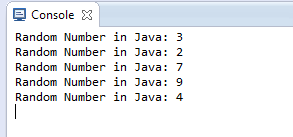# Generate Random Number in Java in a Range

In this tutorial I will teach you how to generate random number in Java in a range.

There are two ways by which Java random number can be generated. The first way is to use nextInt() method of java.util.Random class and second way is to use random() method of java.lang.Math class.

Below I have shared one example for each.

## Generate Random Number in Java in a Range

### java.util.Random

The nextInt() method generates random number in a range. Below example will generate random number in between 1 and 10. Here 1 is inclusive and 10 is exclusive.

Output### java.lang.Math

The random() method generates a random number in between 0.0 and 1.0. Here 0.0 is inclusive and 1.0 is exclusive. In below example I have multiplied the result with 10 and type casting it into int to get random number in between 1 and 10.

OutputIf you found anything incorrect or have any doubts regarding above Java random number tutorial then comment below.

### 2 thoughts on “Generate Random Number in Java in a Range”

1.You can add a third alternative making use of org.apache.commons.lang3.RandomUtils:
RandomUtils.nextInt(1, 10); // 1 is inclusive, 10 exclusive

2.